# Power Function

## power function

[′pau̇·ər ‚fəŋk·shən]
(mathematics)
A function whose value is the product of a constant and a power of the independent variable.
(statistics)
The function that indicates the probability of rejecting the null hypothesis for all possible values of the population parameter for a given critical region.

## Power Function

the function f(x) = xa, where a is a fixed number. Usually only real values of xa are considered for real values of the base x and exponent a. The function has real values for all x > 0. If a is a rational number with an odd denominator, the function also has real values for all x < 0. If, however, a is a rational number with an even denominator or if a is irrational, then xa has no real values for any x < 0. When x = 0, the power function is equal to 0 for all a > 0 and is undefined for a < 0; 00 has no definite meaning.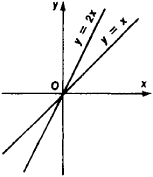Figure 1

The power function is single-valued in the domain of real numbers except when a is a rational number that can be represented by an irreducible fraction with an even denominator. When a is such a rational number, the function is double-valued and assumes values equal in absolute value but opposite in sign for the same value of the argument x > 0. Only the nonnegative value of the function is generally considered in this case. For x > 0, xa is increasing if a > 0 and decreasing if a < 0.

The power function is continuous and differentiable at all points of its domain of definition except at the point x = 0 when 0 < a < 1 (continuity is preserved in this case, but the derivative becomes infinite). The derivative is given by the equation (xa)’ = axa-1. Furthermore,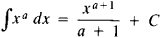when a ≠ – 1, and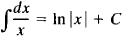These two equations hold in any interval in the domain of definition of the integrand.

Functions of the form y = cxa, where c is a constant, play an important role in pure and applied mathematics. When a = 1, such functions express a direct proportion, and their graphs are lines that pass through the origin (see Figure 1). When a = – 1, the functions express an inverse proportion; their graphs are equilateral hyperbolas whose center is at the origin and whose asymptotes are the coordinate axes (see Figure 2).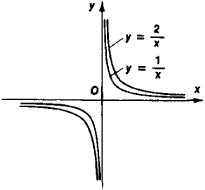Figure 2

Many laws of physics are expressed mathematically by functions of the form y = cxa (see Figure 3). For example, y = ex2 expresses the law of uniformly accelerated or decelerated motion. Here, y is the distance traveled, x is the time, and 2c is the acceleration; the initial distance and speed are both 0.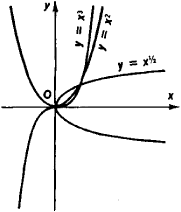Figure 3

In the complex domain the power function za is defined for all z ≠ 0 by the formula

(*) za = exp a Ln z = exp a(lnǀzǀ + i argz + 2kπi)

where k = 0, ±1, ±2, .... If a is an integer, za is single-valued:

za = ǀzǀa exp ia arg z

Suppose a is rational—that is, a = p/q, where p and q are relatively prime. Then za takes on q distinct values:

(za)k = ǀ z ǀak exp ia arg z

Here, ∊k = exp 2kπi/q are the q th roots of 1: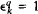, and k = 0, 1,. . . , q – 1. If a is irrational, then za has infinitely many values: the factor exp 2kπia takes on distinct values for distinct k. When a is complex, za is defined by the same formula (*). For example,

zi = exp i(In ǀzǀ + i arg z + 2kπi)

= exp (i In ǀzǀ – argz – 2kπ)

In particular, ii = exp (–π/2 – 2kπ), where k = 0, ±1, ±2, ...

The principal value (za)0 of a power function is the function’s value when k = 0 if – π < arg z ≤ π (or 0 ≤ arg z < 2π). Thus,

(za)0 = ǀzaǀ exp ia argz

For example, (i)0 = exp –π/2.

References in periodicals archive ?
Figures 3 4 and 5 present the results for linear exponential and power function respectively.
The smart meter's third power function is its ability to safely connect and disconnect the remote customer's power during a shortage of power sources.
For any positive integer n, the Smarandache power function SP(n) is defined as the smallest positive integer m such that n | [m.sup.m], where m and n have the same prime divisors.
For these continuous densities--the power function, three versions of the Weibull, two versions of the lognormal, and the gamma--the inflation of Type I error rates is as pronounced, or more so, as that of the exponential distribution.
Schillig showed the Maxx, a convertible sectional with a power function similar to a hospital bed.
The program displays performance characteristics-in the form of OPSpecs charts, critical error graphs, and power function graphs--for various sets of QC rules.
Once the candidate QC procedures are selected, the "options" menu in the "parameters" window is used to choose power function graphs, critical error graphs, or OPSpecs (operational process specifications) charts.
The board features five Gigabit Ethernet ports that support LAN Bypass, a USB WLAN interface, four S-ATA ports for data logging or signature, serial ports for console redirection with an LCM power function, plus several other features.
Abstract For any positive integer n, let SP(n) denotes the Smarandache power function, and 0(n) is the Enter totient function.
Figure 5A displays the power function curves for the [1.sub.2.18s] rule ([P.sub.fr] = 0.058) and the [bar.X](2.49)/[R.sub.4s] rule ([P.sub.fr] = 0.017) for two control samples.
2,304 LEGO Power Function motors join forces to power LEGO TEchnics super sports car, which weighs 1,500 kg.

Site: Follow: Share:
Open / Close